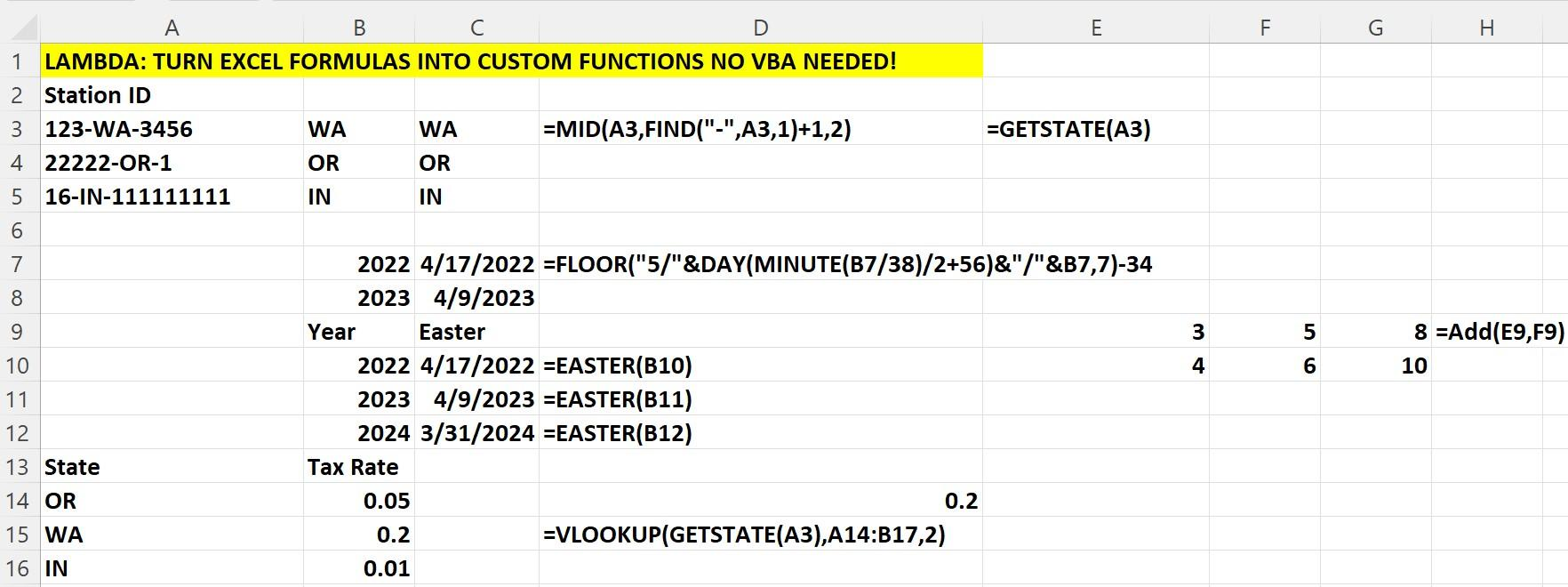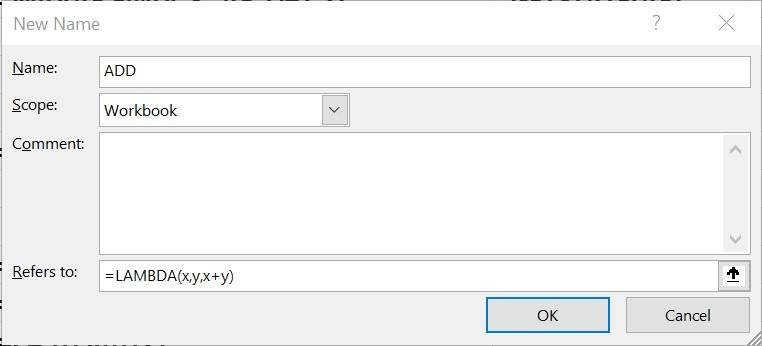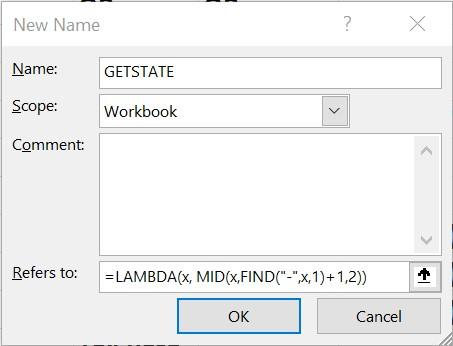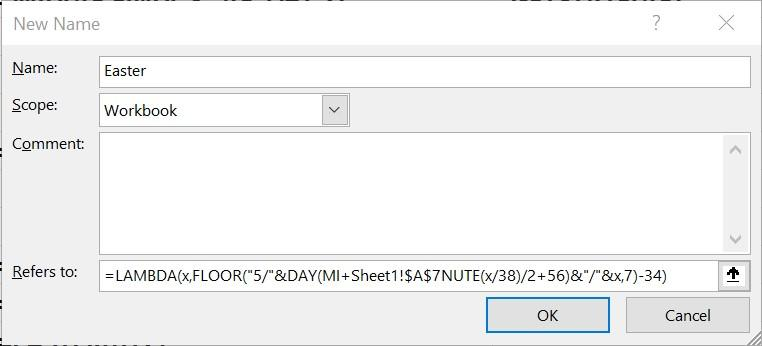# Excel LAMBDA functions to the rescue!Many of you have used Custom Functions in Visual Basic for Applications (VBA). For example, to price a European call option, an investment professional might enter the formula =BSCALL(40,45,.5,.2,.05) to compute a Black-Scholes price for a six-month call option in the following situation:

• The stock is currently selling for \$40.
• The exercise price of the call option is \$45.
• The option’s duration is six months (.5 years).
• The stock’s annual volatility is 20%.
• The current interest rate is 5%.

The function BSCALL has been coded in Excel’s VBA by a very smart person to compute the price of a call for any combination of the five input values: [Value 1, Value 2, Value 3, Value 4, Value 5]. For more in-depth details on the BSCALL function, see Simon Benninga’s great book “Financial Modeling.”

If you have Office 365, the new Excel LAMBDA function, which was introduced in late 2020, allows you to create custom functions without VBA.

In this article, we will use three examples to show you how easy it is to use LAMBDA to create new functions. Our work is in the workbook Lambda.xlsx, as shown in Figure 1.Figure 1. Examples of Excel LAMBDA functions

## Adding two numbers with Excel LAMBDA functions

We want to create a simple function, Add, which will add two numbers. All we need to do is select Define Name from the Defined Name group on the Formula tab and fill in the New Name dialog box as shown in Figure 2.Figure 2. A function to add two numbers

Our formula, =LAMBDA(x,y,x+y), tells Excel that when we type “Add” and give Excel two values, Excel should add them up. For example, entering the formula =Add(E9,F9) in cell G9 returns 3+5 = 8 in cell G9. By the way, you can view, edit or delete your LAMBDA functions by going to the Name Manager in the Defined Names group on the Formula tab.

## Returning a state abbreviation from a text string with Excel LAMBDA functions

In cells A3:A5 we entered code names for three radio stations. After the first hyphen, there is a two-character state abbreviation. We want to write a LAMBDA function, GETSTATE, that will return the state abbreviation. The following Excel formula =MID(A3,FIND("-",A3,1)+1,2) returns the two characters after the first hyphen. To create a LAMBDA function named GETSTATE that returns the state abbreviation, simply fill in the New Name Dialog Box as shown in Figure 3:Figure 3. Returning state abbreviation

Entering the formula =GETSTATE(A3) in cell G3 returns the state WA for the radio station ID shown in cell A3 of the workbook.

## Computing the date of Easter with Excel LAMBDA functions

I searched for an Excel formula that would compute the date of Easter for year x and found the following formula:

=FLOOR("5/"&DAY(MINUTE(x/38)/2+56)&"/"&x,7)-34.

Of course, we want a function EASTER that would compute, say, for the year in cell B10 the date of Easter with the formula =EASTER(B10). To create this function, complete the New Name Dialog Box as shown in Figure 4.Figure 4. Computing the date of Easter

Copying the formula =EASTER(B10) from cell C10 to C11:C12 returns the date of Easter for the years 2022-2024. You might want to search for similar formulas to compute the start of Passover or Ramadan.

As I’ve demonstrated, Excel’s LAMBDA functions can help save time by creating your own custom shortcuts. Next month, we will show you how Excel’s DATA CONSOLIDATE capability makes it easy to summarize data in multiple locations.

For more tips and tricks, take a Becker CPE Microsoft Excel course.

### Now Leaving Becker.com

You are leaving the Becker.com website. Once you click “continue,” you will be brought to a third-party website. Please be aware, the privacy policy may differ on the third-party website. Adtalem Global Education is not responsible for the security, contents and accuracy of any information provided on the third-party website. Note that the website may still be a third-party website even the format is similar to the Becker.com website.